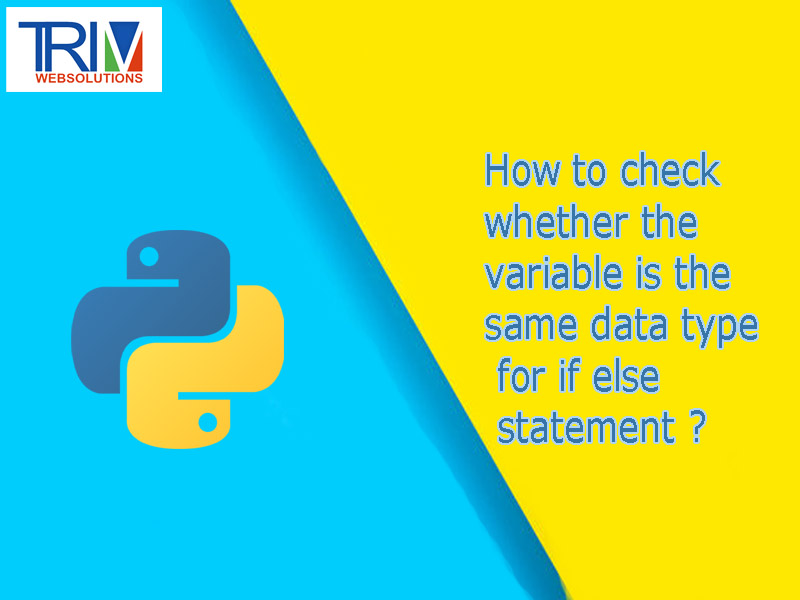Quick Inquiry

# How to check whether the variable is the same data type for if else statement in python ?There are multiple methods that could be used to determine data types in this situation - however, each is slightly different. Python has the `Type()` function. Though this function has three parameters `type(object, bases, dict)`, only one is required, the object. If you would like to learn about the other parameters, You can use an if else statement to determine if the object type is a string -

``` ```import sys #to be used for error message

user_input = input("prompt")
input_type = Type(user_input)

if input_type == int:
do_whatever()
else:
sys.exit("Please try again with an integer input") ``````

However, due to the nature of the input() function, this will not work in this instance, as input() always returns a string - however, the other two methods are still valid.

Another method is to use an Exception, as mentioned by Sruthi V. When converting to an integer, if a string is inputted, an error will occur.

``` ```try:
user_input = input("prompt")
user_input = int(user_input)
except ValueError:
print("Please run the program again and enter an integer") ``````

Finally, you can use the `isinstance()` function - essentially an alternative to the Type function. `isinstance()` takes two values, `(object, type)`. A bool of true or false will be returned, depending on if the type of object is the same as the parameter `type`.

``` ```import sys

user_input = input("prompt")
if isinstance(user_input, int):
do_whatever()
else:
sys.exit('Run the program again with an integer input') ``````

However, you already check for int at the beginning of your file - an error will occur if any value that is not an int is detected. Therefore, in your specific situation, there is no need to check if the value is int. You may use exceptions to print custom messages on input of a non-integer value, but checking if the value is an int again is repetitive, and uses unnecessary resources.